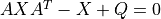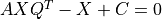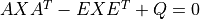# control.matlab.dlyap¶

control.matlab.dlyap(A, Q, C=None, E=None, method=None)[source]

Solves the discrete-time Lyapunov equation

X = dlyap(A, Q) solveswhere A and Q are square matrices of the same dimension. Further Q must be symmetric.

dlyap(A, Q, C) solves the Sylvester equationwhere A and Q are square matrices.

dlyap(A, Q, None, E) solves the generalized discrete-time Lyapunov equationwhere Q is a symmetric matrix and A, Q and E are square matrices of the same dimension.

Parameters
• A (2D array_like) – Input matrices for the Lyapunov or Sylvestor equation

• Q (2D array_like) – Input matrices for the Lyapunov or Sylvestor equation

• C (2D array_like, optional) – If present, solve the Sylvester equation

• E (2D array_like, optional) – If present, solve the generalized Lyapunov equation

• method (str, optional) – Set the method used for computing the result. Current methods are ‘slycot’ and ‘scipy’. If set to None (default), try ‘slycot’ first and then ‘scipy’.

Returns

X – Solution to the Lyapunov or Sylvester equation

Return type

2D array (or matrix)

Notes

The return type for 2D arrays depends on the default class set for state space operations. See `use_numpy_matrix()`.